0%

# 学习提示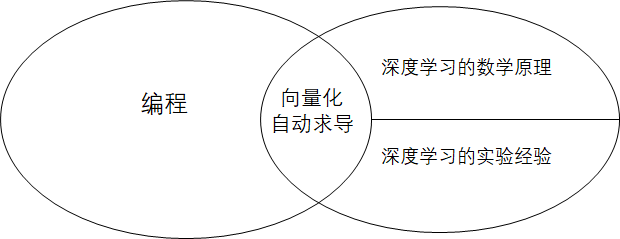# 课堂笔记

## 本节课的目标

### 符号标记

$(x, y)$ 是一个训练样本。其中，$x$ 是一个长度为 $n_x$ 的一维向量，即 $x \in \mathcal{R}^{n_x}$。$y$ 是一个实数，取0或1，即$y \in {0, 1}$。取0表示问题的的答案为“否”，取1表示问题的答案为“是”。

## 逻辑回归的公式描述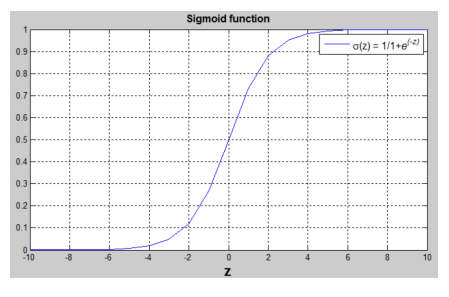## 优化算法——梯度下降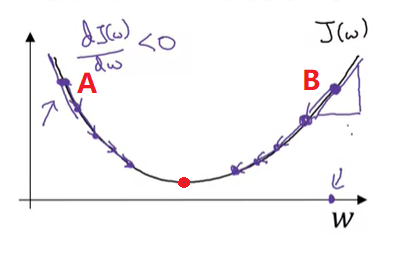## 前置知识补充

### 计算图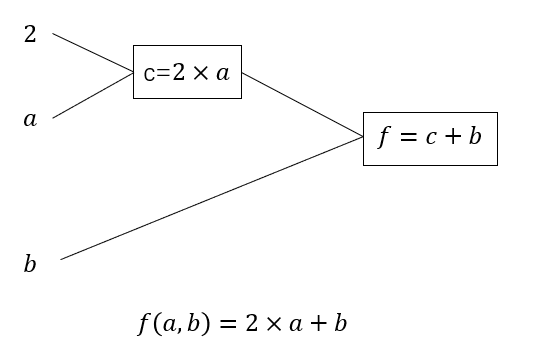### 利用计算图对逻辑回归求导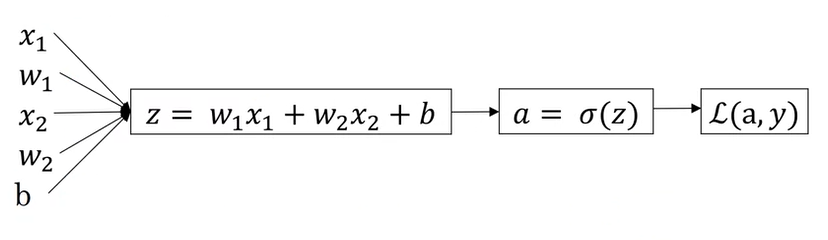$\frac{dL}{dw_i}, \frac{dL}{db}$就是我们要的梯度了，用它们去更新原来的参数即可。值得一提的是，这里的梯度是对一个样本而言。对于全部$m$个样本来说，本轮的梯度应该是所有样本的梯度的平均值。后面我们会学习如何对所有样本求导。

### Python 向量化计算

1. 对$m$个样本循环处理
2. 对$n_x$个权重$w_i$与对应的$x_i$相乘

Python 的 numpy 库提供了向量化计算的接口。比如以下是向量化的例子：

numpy 允许一种叫做“广播”的操作，这种操作能够完成不同形状数据间的运算。

## 向量化计算前向和反向传播

$dz$ 是 $\frac{dJ}{dz}$的简写，其他变量同理。编程时也按同样的方式命名。

np.dot实现了求向量内积或矩阵乘法，np.sum实现了求和，np.mean实现了求均值。

## 总结

• 什么是二分类问题。
• 如何对建立逻辑回归模型。
• Sigmoid 函数 $\sigma(z)=\frac{1}{1 + e^{-z}}$
• 误差函数与损失函数
• 逻辑回归的误差函数：$L(\hat{y}, y)=-(y \ log\hat{y} + (1-y) \ log(1-\hat{y}))$
• 用梯度下降算法优化损失函数
• 计算图的概念及如何利用计算图算梯度

• 了解numpy基本知识
• resize
• .T
• exp
• dot
• mean, sum
• 用numpy做向量化计算
• 实现逻辑回归
• 对输入数据做reshape的预处理
• 用向量化计算算$\hat{y}$及参数的梯度
• 迭代优化损失函数

# 代码实战

## 程序设计

• 数据预处理
• 定义网络结构
• 定义损失函数
• 定义优化策略
• 用训练pipeline串联起网络、损失函数、优化策略
• 测试模型精度

## 数据收集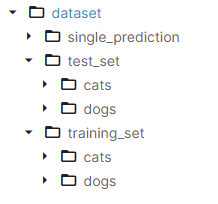## 数据预处理

1. 这个函数有一个参数data_num，表示我们要读取data_num张猫+data_num张狗的数据。原数据集有上千张图片，直接读进内存肯定会把内存塞爆。为了实现上的方便，我加了一个控制数据数量的参数。在这个项目中，我只用了800张图片做训练集。
2. 原图片是很大的，为了节约内存，我把所有图片都变成了input_shape=(224, 224)的大小。

Python里， * 10可以把列表复制10次。

## 网络结构

1. resize_input：由于图片张量的形状是[h, w, c]（高、宽、颜色通道），而网络的输入是一个列向量，我们要把图片张量resize一下。
2. sigmoid: 我们要用numpy函数组合出一个sigmoid函数。

array = array.reshape(a, b) 等价于 array.resize(a, b)。但是，reshape的某一维可以写成-1，表示这一维的大小让程序自己用除法算出来。比如总共有a * b个元素，调用reshape(-1, a)-1的那一维会变成b

## 测试

y_hat > 0.5是有实际意义的：在二分类问题中，如果网络输出图片是小猫的概率大于0.5，我们就认为图片就是小猫的图片；否则，我们认为不是。